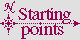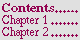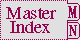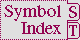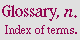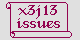#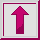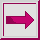Variable *, **, ***

Value Type:

an object.

Initial Value:

Description:

The variables *, **, and *** are maintained by the Lisp read-eval-print loop to save the values of results that are printed each time through the loop.

The value of * is the most recent primary value that was printed, the value of ** is the previous value of *, and the value of *** is the previous value of **.

If several values are produced, * contains the first value only; * contains nil if zero values are produced.

The values of *, **, and *** are updated immediately prior to printing the return value of a top-level form by the Lisp read-eval-print loop. If the evaluation of such a form is aborted prior to its normal return, the values of *, **, and *** are not updated.

Examples:

```(values 'a1 'a2) =>  A1, A2
'b =>  B
(values 'c1 'c2 'c3) =>  C1, C2, C3
(list * ** ***) =>  (C1 B A1)

(defun cube-root (x) (expt x 1/3)) =>  CUBE-ROOT
(compile *) =>  CUBE-ROOT
(setq a (cube-root 27.0)) =>  3.0
(* * 9.0) =>  27.0
```

Affected By:

``` *   ==  (car /)